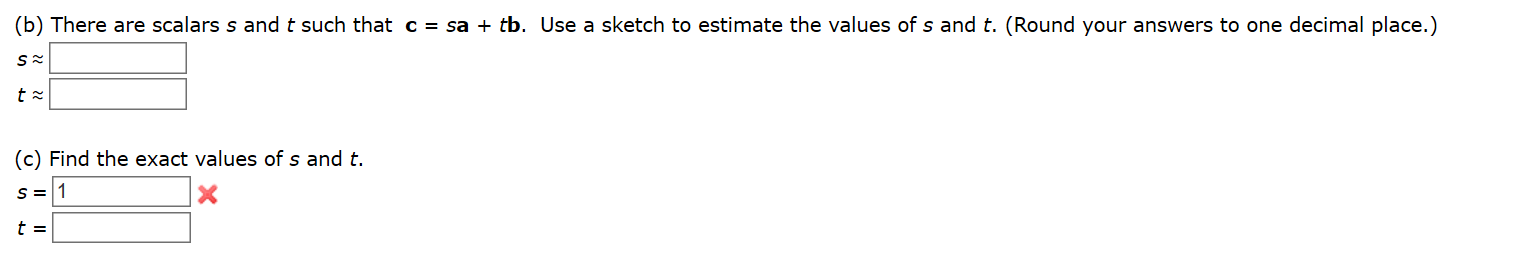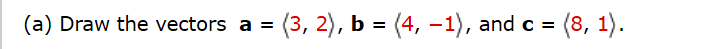# (b) There are scalars s and t such thatc = sa + tb.Use a sketch to estimate the values of s and t. (Round your answers to one decimal place.)(c) Find the exact values of s and t. (3, 2), b = (4, –1), and c ==(8, 1).(a) Draw the vectors a =

Question
10 viewshelp_outlineImage Transcriptionclose(b) There are scalars s and t such that c = sa + tb. Use a sketch to estimate the values of s and t. (Round your answers to one decimal place.) (c) Find the exact values of s and t. fullscreenhelp_outlineImage Transcriptionclose(3, 2), b = (4, –1), and c = =(8, 1). (a) Draw the vectors a = fullscreen
check_circle

Step 1

Hey, since there are multiple questions posted, we will answer first question. If you want any specific question to be answered then please submit that question only or specify the question number in your message.

The given vectors are

...

### Want to see the full answer?

See Solution

#### Want to see this answer and more?

Solutions are written by subject experts who are available 24/7. Questions are typically answered within 1 hour.*

See Solution
*Response times may vary by subject and question.
Tagged in